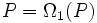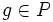# Omega-1 of odd-order p-group is coprime automorphism-faithful

This article gives the statement, and possibly proof, of a particular subgroup of kind of subgroup in a finite group being coprime automorphism-faithful. In other words, any non-identity automorphism of of the whole group, of coprime order to the whole group, that restricts to the subgroup, restricts to a non-identity automorphism of the subgroup.
View other such statements

## Statement

Suppose$p$ is an odd prime, and$P$ is a finite$p$-group (i.e., a Group of prime power order (?)). Let$\Omega_1(P)$ be the first omega-subgroup of$P$, i.e.:$\Omega_1(P) = \langle x \mid x^p = e \rangle$.

Then,$\Omega_1(P)$ is a Coprime automorphism-faithful subgroup (?) of$P$: if$\sigma$ is a non-identity automorphism of$P$ of order relatively prime to$p$, then the restriction of$\sigma$ to$\Omega_1(P)$ is also a non-identity automorphism.

## Related facts

### Failure for the prime two

For the prime two, the quaternion group is a group where$\Omega_1$ is not coprime automorphism-faithful: for instance, any non-identity inner automorphism has order two, but acts as the identity on$\Omega_1$, which is equal to the center.

However, a partial equivalent is true at the prime two:

## Proof

Given: An odd prime number$p$, a finite$p$-group$P$, an automorphism$\sigma$ of$P$ such that the order of$\sigma$ is relatively prime to$p$, and such that the restriction of$\sigma$ to$\Omega_1(P)$ is the identity map.

To prove:$\sigma$ is the identity map on$P$.

Proof: We prove the claim by induction on the order of the group, so we can assume that the result is true for every proper subgroup of$P$. Also, we assume that$\sigma$ is a non-identity automorphism and derive a contradiction.

First, note that for$Q \le P$,$\Omega_1(Q) \le \Omega_1(P)$. Since$\sigma$ acts as the identity on$\Omega_1(P)$, we conclude that if$Q$ is$\sigma$-invariant,$\sigma$ acts as the identity on$Q$. Now, if$\sigma$ is a non-identity automorphism, apply fact (1) for the element$\sigma$ and the cyclic group generated by$\sigma$, acting on$P$. The upshot:$P$ is either elementary Abelian or special. If$P$ is Abelian, and$\sigma$ acts nontrivially on$P/P'$.

The case that$P$ is elementary Abelian can be eliminated, because in that case$P = \Omega_1(P)$, so$\sigma$ being the identity on$\Omega_1(P)$ forces it to be the identity on$P$. Thus, the only case left is that$P$ is a special group.

By fact (2), the$p$-power map is an endomorphism, so for any$g \in P$,$(g^{-1}\sigma(g))^p = g^{-p}\sigma(g^p)$. Now,$g^p \in Z(P)$ since$P/Z(P)$ is elementary Abelian. Further, since$P$ is special$Z(P)$ itself is elementary Abelian, so$Z(P) \le \Omega_1(P)$, and consequently$\sigma$ acts as the identity on$Z(P)$. Thus,$g^p = \sigma(g^p)$, so$(g^{-1}\sigma(g))^p = e$.

Thus, for any$g \in P$,$g$ and$\sigma(g)$ are in the same coset of$\Omega_1(P)$. Thus,$\sigma$ acts as the identity on$P/\Omega_1(P)$. By assumption,$\sigma$ also acts as the identity on$\Omega_1(P)$. Combining this with fact (3), we obtain that$\sigma$ is the identity map.